# Reynold's Transport Theorem

• kev931210

## Homework Statement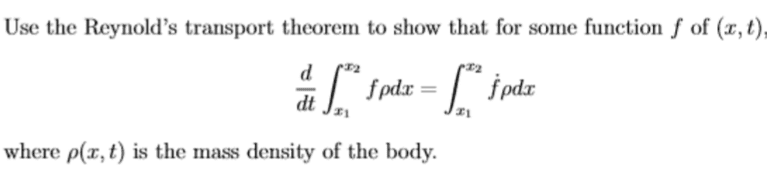## Homework Equations

one dimensional Reynold's transport theorem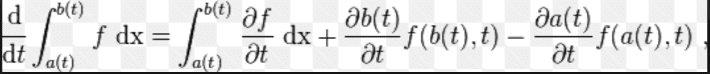## The Attempt at a Solution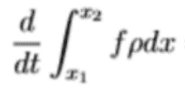[/B]

I started with this equation, and tried to expand it using the equation given in #2.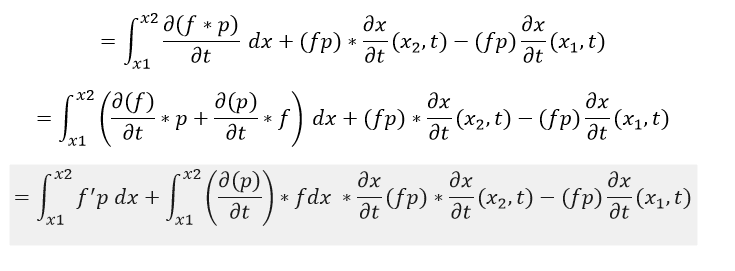This is the farthest I have gotten so far. I got stuck from here. I do not know how to get from the shaded equation to the equation below.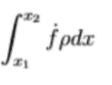help please .. I just want to pass (and learn)helpppppppppppppppppppppppppppppppppppppppppppppppppppppppppppppppppppppppppppppppppppppppppppppppppppppppppppppppppppppppppppppppppppppppppppppppppppppppppppppppppppppppppppppppppppppppppppppppppppppppppppppppppppppppppppppppppppppppppppppppppp

Substitute ##v_1=\frac{dx_1}{dt}## and ##v_2=\frac{dx_2}{dt}## into your second equation. What does that give you? Incidentally, the ##f\rho##'s in this equation should be evaluated at 1 and 2.

•kev931210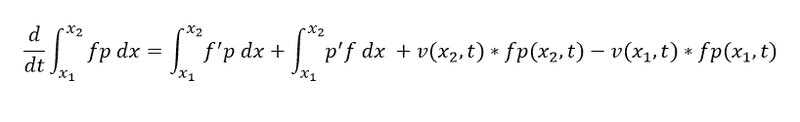Sorry, there was some typo in my question. So, I ended up with the above equation using your advice, but it doesn't seem to get me any further. Both f and p are functions of (x,t), so it's hard for me to simplify easily. Is there any relation that can let me convert the above equation to the below equation?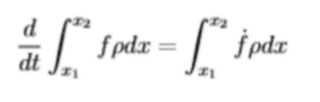#### Attachments

Maybe it would help if I wrote your expression using LateX, which you should learn from the PF tutorial:

$$\int_{x_1}^{x_2}{\left(\rho\frac{\partial f}{\partial t}+f\frac{\partial \rho}{\partial t}\right)dx}+(\rho v f)_{x_2}-(\rho vf)_{x_1}$$

Does this give you any ideas?

•kev931210
No .. sorry I'm not an advanced student in Physics. Is there any physics theory or mathematical trick I can use from that point? I have no clue what to do from that point. I was stuck there for long time.

No .. sorry I'm not an advanced student in Physics. Is there any physics theory or mathematical trick I can use from that point? I have no clue what to do from that point. I was stuck there for long time.
$$(\rho v f)_{x_2}-(\rho vf)_{x_1}=\int_{x_1}^{x_2}{\frac{\partial (\rho vf)}{\partial x}dx}$$

•kev931210
Sorry, I could not find the tutorial, and ended up not using latex to write the equations.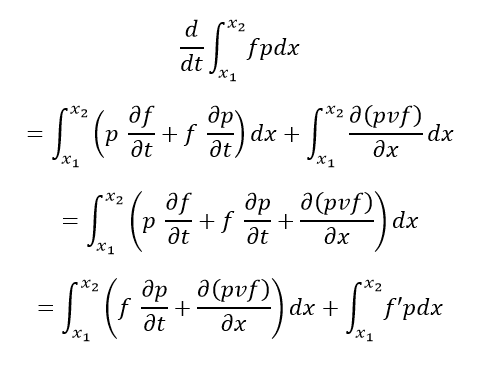In order to derive the equation given by the question, the following equation needs to be true: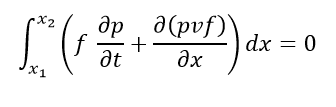If f is independent of x, I can easily extract f, and prove the above equation by using the mass balance equation. However, f is a function of x and t, so I am not so sure how I can derive the above equation...

•kev931210
Sorry, I could not find the tutorial, and ended up not using latex to write the equations.

View attachment 99882
The f' they are referring to is not ##=\partial f/\partial t##. It is $$f'=\frac{\partial f}{\partial t}+v\frac{\partial f}{\partial x}$$

•kev931210
Oh... that makes sense. Thank you so much!

Oh... that makes sense. Thank you so much!
So you got it now?

Yes I got it now. It simplified quite easily with your help. Thank you.